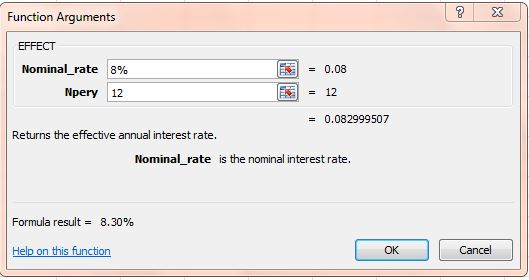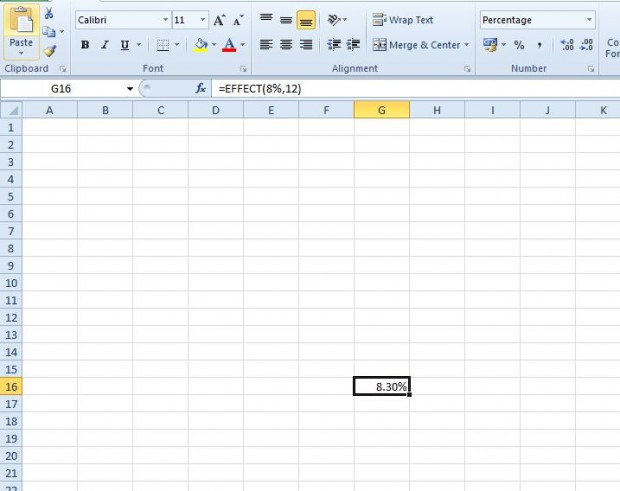# How to use EFFECT function in Excel spreadsheets [Tip]

A nominal interest rate for a loan is one that does not factor in compound interest. However, as interest rates are compounded monthly, or quarterly, the effective rate of loan interest is slightly higher. To find the effective rate of interest, you can add the EFFECT function to Excel 2010/13 spreadsheets. The EFFECT function’s syntax is EFFECT(nominal_rate, npery).

For example, you could find the effective rate of interest for an 8% loan with monthly instalments. Open an Excel spreadsheet, and click the fx button. Then you can select EFFECT from the Insert Function window.You can enter the nominal rate of interest and the number of compounding periods in the window above. Enter 8% in the Nominal_rate field. Below the Nominal_rate field enter 12, the number of compounding periods, in the Npery field. Click OK to close window and add the EFFECT function to spreadsheet.The function returns a value 0.083% as in the shot above. That’s an effective interest rate of 8.3%. You can adjust the format of the cell to percent by right-clicking it and selecting Format Cells. Then click the Format tab and select Percentage.

So with this function you can now find the effective interest rate (APY) of loans. This video also shows how to add the EFFECT function to Excel 2010/13 spreadsheets.